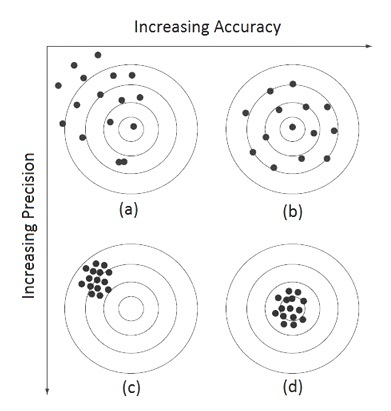# Accuracy and Precision###### By MATLAB Helper

The concept of different types of errors associated with both calculations and measurements are introduced in this lesson.

`lang: en_US`

Tip: Participate in Numerical Methods Course Certification Quiz to test your knowledge. Scroll to the bottom and know more!

Nowadays, computers are great tools in numerical computation, which plays an essential part in solving real-life mathematical, physical, and engineering problems. However, without a fundamental understanding of engineering problems, it will be of no use.

The first topic that we are going to see in numerical methods would be error analysis using MATLAB. The first type of error is an absolute error. The errors associated with both calculations and measurements can be characterized by their accuracy and precision. Accuracy refers to how closely a computed or measured value agrees with the true value. Precision refers to how closely individual computer measured values agree with each other.Figure 1: (a) inaccurate and imprecise, (b) accurate and imprecise,(c) Inaccurate and precise, (d) accurate and precise

These concepts can be illustrated graphically using an analogy from target practice. The bullet holes on each target in Fig. 1 can be thought of as the predictions of a numerical technique, whereas the bull’s-eye represents the truth. Inaccuracy (also called bias) is defined as a systematic deviation from the truth. Thus, although the shots in Fig. 1are more tightly grouped than in Fig. 1a, the two cases are equally biased because they are both centered on the upper left quadrant of the target. Imprecision (also called uncertainty), on the other hand, refers to the magnitude of the scatter. Therefore, although Fig.1and 1are equally accurate (i.e., centered on the bull’s-eye), the latter is more precise because the shots are tightly grouped.

## Various Kinds of Computing Errors

 Absolute Error The absolute difference between the true value and the approximation. Relative Error Absolute error divided by the absolute of true value Round off Error Caused by representing/storing numeric data in finite bits. Truncation Error Inaccuracies are introduced by the truncation of an infinite process. Numerical Error Round off error + Truncation error

We hope this lesson helped you in getting knowledge about accuracy and precision. In the upcoming lesson, we have covered Absolute error, an error in numerical analysis.